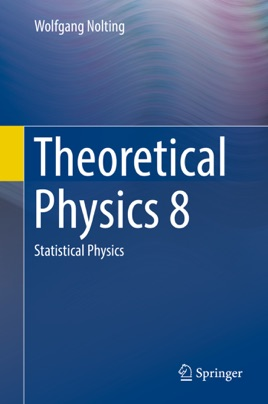• \$84.99

## Publisher Description

This textbook offers a clear and comprehensive introduction to statistical physics, one of the core components of advanced undergraduate physics courses. It follows on naturally from the previous volumes in this series, using methods of probability theory and statistics to solve physical problems. The first part of the book gives a detailed overview on classical statistical physics and introduces all mathematical tools needed. The second part of the book covers topics related to quantized states, gives a thorough introduction to quantum statistics, followed by a concise treatment of quantum gases.

Ideally suited to undergraduate students with some grounding in quantum mechanics, the book is enhanced throughout with learning features such as boxed inserts and chapter summaries, with key mathematical derivations highlighted to aid understanding. The text is supported by numerous worked examples and end of chapter problem sets.